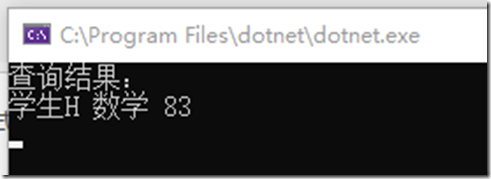Categories

# C # expression trees Lambda extension (four)

I. Introduction

This article was originally written calculation behind the need to use the time, but since it is written to the expansion of the expression, it is finished with it.

See this title there is a doubt, Lambda expressions has always been an expression tree, but also how the expansion? Then take a look at the following, you know.

Expressions Series Catalog

C # expression trees to explain (a)

C # expression tree traversal (b)

C # expression trees Sorting Expansion (c)

C # expression trees Lambda extension (four)

Two, Lambda extension

Here the first busy to answer the above questions, we look at such a scenario.

Requesting a page, with which a number of query conditions, following a request class

```public class ScoreRequest
{
public string CourseName { get; set; }
public string StudentName { get; set; }
}
```

Requests for access to the course name and the name of the student matching data

We have more than one data source data source example

Data source class```public class ScoreClass
{
public string CourseName { get; set; }
public string StudentName { get; set; }
public decimal Score { get; set; }
}
```

View Code```var datas = new List();
{
CourseName = "mathematics",
StudentName = "A student",
Score = 60
});
{
CourseName = "mathematics",
StudentName = "Student B",
Score = 65
});
{
CourseName = "mathematics",
StudentName = "Student C",
Score = 70
});
{
CourseName = "mathematics",
StudentName = "Student D",
Score = 75
});
{
CourseName = "mathematics",
StudentName = "Student E",
Score = 80
});
{
CourseName = "mathematics",
StudentName = "Students F",
Score = 81
});
{
CourseName = "mathematics",
StudentName = "Students G",
Score = 82
});
{
CourseName = "mathematics",
StudentName = "H students",
Score = 83
});
{
CourseName = "mathematics",
StudentName = "Students I",
Score = 84
});
```

View Code

Well now we can query data

```var request = new ScoreRequest()
{
CourseName = "number",
StudentName = "H"
};
var resultDatas = datas.Where(e => e.CourseName.Contains(request.CourseName) && e.StudentName.Contains(request.StudentName))
.ToList();```

If the query object inside CourseName and StudentName fields are worth, then write no problem. If there is no value, then the final data is not accurate.

If you are directly put together sql statements, we can use to judge if (String.IsNullOrEmpty ()), but now a judge, how to piece together Lambda expressions do?

So we need to extend the Lambda expressions, so that he supported such a case. That the above problems, it is no longer dedicated to answer it! ! ! !

Create a LambdaExtension class code is as follows

```public static class LambdaExtension
{
public static Expressionbool>> True() { return param => true; }
public static Expressionbool>> False() { return param => false; }
public static Expressionbool>> And(this Expressionbool>> first, Expressionbool>> second)
{
return first.Compose(second, Expression.AndAlso);
}
public static Expressionbool>> Or(this Expressionbool>> first, Expressionbool>> second)
{
return first.Compose(second, Expression.OrElse);
}
private static Expression Compose(this Expression first, Expression second, Func merge)
{
var map = first.Parameters
.Select((f, i) => new { f, s = second.Parameters[i] })
.ToDictionary(p => p.s, p => p.f);
var secondBody = PFTParameterExtension.ReplaceParameters(map, second.Body);
return Expression.Lambda(merge(first.Body, secondBody), first.Parameters);
}

private class PFTParameterExtension : ExpressionVisitor
{

public PFTParameterExtension()
{

}

public PFTParameterExtension(Dictionary map)
{
this.map = map ?? new Dictionary();
}

///
/// parameter substitution
///
/// The map.
/// The exp.
/// Expression
public static Expression ReplaceParameters(Dictionary map, Expression exp)
{
return new PFTParameterExtension(map).Visit(exp);
}

protected override Expression VisitParameter(ParameterExpression p)
{
ParameterExpression replacement;
if (map != null && map.Count > 0 && map.TryGetValue(p, out replacement))
{
p = replacement;
}
return base.VisitParameter(p);
}

}

}```

There is a privatization of the expression tree visitors, his role is mainly used to synchronize Lambda expressions inside the parameters.

The following is a way to call

```            var expression = LambdaExtension.True();
if (!string.IsNullOrWhiteSpace(request.CourseName))
expression = expression.And(e => e.CourseName.Contains(request.CourseName));
if (!string.IsNullOrWhiteSpace(request.StudentName))
expression = expression.And(et => et.StudentName.Contains(request.StudentName));

var resultDatas = datas.Where(expression.Compile())
.ToList();
Console.WriteLine(\$"Query results: \ n {string.Join ("\n", resultDatas.Select(e => \$"{e.StudentName} {e.CourseName} {e.Score}"))}");
```

where conditions inside only with the commission, and our expression is Lambda expressions, it is necessary to Compile commissioned to compile.

operation result:A closer look at the code, the first parameter is inside And condition “e”, the second parameter is inside et condition, with which a Lambda expression (there is only one parameter), the parameter must be the same, so the class LambdaExtension , the merger of the two Lambda expressions, it needs to be combined into one parameter.

After this expansion, we can according to our actual situation, put together an expression that you need, to get the results we want.

Third, the summary

Explain aspects of the expression tree, finally came to an end. He has no such after writing articles, now that writing articles or really tiring, this year’s three-day Mid-Autumn Festival, considered all of the park to the blog. But three days to explain the content, basically put behind Dapper extension technologies are needed to pave the way, we will continue to explain to the back of the ORM. In fact, I did not write a blog post, the snail will go to the list and sort out the relevant knowledge, which also benefited let snails, snail blog also hope to be able to help the Friends of Park, which is called the “gifts of roses, hand left lingering fragrance “it.